What is Probability in Statistics?

Probability Theory

Because data used in statistical analyses often involves some amount of "chance" or random variation, understanding probability helps us to understand statistics and how to apply it.

Key Terms

o       Random experiment

o       Outcome

o       Event

o       Sample space

o       Mutually exclusive

o       Random variable

o       Probability

o       Complement

o       Union

o       Intersection

Objectives

o       Recognize and understand the basic terms associated with probability theory

o       Learn how probability is related to statistics

o       Perform simple calculations related to probability

Probability and statistics are actually quite extensively linked. For instance, when a scientist performs a measurement, the outcome of that measurement has a certain amount of "chance" associated with it: factors such as electronic noise in the equipment, minor fluctuations in environmental conditions, and even human error have a random effect on the measurement. Often, the variations caused by these factors are minor, but they do have a significant effect in many cases. As a result, the scientist cannot expect to get the exact same measurement result in every case, and this variation requires that he describe his measurements statistically (for instance, using a mean and standard deviation). Likewise, when an anthropologist considers a small group of people from a larger population, the results of his study (assuming they involve numerical data) will involve some random variations that he must take into account using statistics.

This type of link between probability (randomness or "chance") and statistics applies to a wide variety of fields that deal with numbers. It therefore behooves us to present some of the basic aspects of probability theory as they relate to statistics.

Probability Terms

Although the concept of randomness (or chance) is difficult to define, we will simply assume that an experiment (or observation) whose outcome cannot be predicted is a random experiment. The outcome of a random experiment is the result of a single instance of the experiment. A set of possible outcomes is called an event--an event can consist of a single outcome or multiple outcomes. For a particular random experiment, the range of potential outcomes may be limited or unlimited; in either case, we call this range the sample space of the experiment. If two events from a particular sample space have no outcomes in common, then those events are mutually exclusive.

A function that is defined for the sample space of some random experiment and that has a finite probability for each value or interval in that sample space is called a random variable. Of course, to understand the definition of a random variable, we must also know what a "probability" is. Recall that relative frequencies of data values: a number between 0 and 1 that expresses a particular datum's fraction of occurrences in the data set. If we conduct a random experiment a large number of times, then the probability of a particular data value (outcome) is its relative frequency. (Ideally, we would have to conduct the experiment an infinite number of times to truly discover the probability.) We can express the probability that an experiment yields a certain outcome for the random variable X (say, X = a, for example) using the following notation:

P(X = a) = p (where 0 ≤ p ≤ 1)

Consider, for instance, a random variable X that corresponds to the outcome of the roll of a single die. The sample space in this experiment is {1, 2, 3, 4, 5, 6}--these are the only potential outcomes of the experiment. If the die is a "fair die," then each outcome has an equal chance of being rolled. This is to say, for any outcome a (where a can be any number in the sample space),

P(X = a) =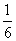Why is this result the case? Because if we roll the die a large number of times, each outcome in the sample space should occur the same number of times. The relative frequency of each outcome must therefore be 1/6, which is the probability cited above.

Occasionally, we will use set notation to describe events. First, we might refer to the complement of an event E. The complement, written EC, is the set of all events in a sample space that are not part of E. Second, we can refer to the union of two events A and B using the following notation: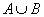The union is simply the set of all outcomes contained in either A or B (or both). Third, we might refer to the intersection of two events A and B as follows: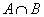The intersection is the set of all outcomes contained in both A and B. If, for instance, we were to consider event E and its complement, EC, for some sample space S, then we can easily find that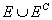is S and that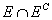is the null (empty) set. These statements are true because E and EC span all of S, but they have no common elements (that is, they are mutually exclusive).

Interested in learning more? Why not take an online Statistics course?

Practice Problem: A statistician conducts a random experiment several times and comes up with the data shown in the table below. Based on this sample data, what should be the statistician's estimate of the probability that the outcome of his next trial of the experiment will be 8?

 Outcome Frequency 1 1 2 2 3 5 4 9 5 13 6 4 7 3 8 2

Solution: We learned that the probability of an event is equal to its relative frequency for a large (infinite) number of trials. Although the data above is limited, the statistician can estimate the probability based on his results. The relative frequency of the outcome 8 is simply the number 2 divided by the total number of trials of the experiment--39 in this case. Thus, the statistician's estimate of the probability of 8 should be approximately 0.05.

Practice Problem: Given events G and H defined below, what are the union and intersection of these events?

G = {1, 3, 4, 5, 9}

H = {1, 3, 7, 8, 10}

Solution: Recall that the union of two events is the event containing all elements in either G or H, or both. The union is then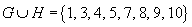The intersection of two events is the event containing all elements in both G and H. Thus,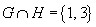Practice Problem: For a six-sided die with faces numbered one through six, what is the complement of the event for which the outcome of a roll is an even number?

Solution: The sample space for the roll of a six-sided die is {1, 2, 3, 4, 5, 6}. Rolling an even number corresponds to the event {2, 4, 6}. The complement of this event are all those outcomes in the sample space that are not part of the event; thus, the complement is all odd numbers--{1, 3, 5}.

Practice Problem: A person must pull a card at random from a standard deck of 52 playing cards. If event A is defined as the person pulling a diamond and event B is defined as the person pulling a spade, determine whether events A and B are mutually exclusive.

Solution: We defined mutually exclusive as the case where two events do not share any outcomes in common. Because there are no cards in the deck that are both a diamond and a spade, events A and B must be mutually exclusive.

Basic Rules of Probability

Now that we have introduced some of the terms associated with probability, we can consider some basic rules. First, recall that the probability of an outcome is equal to its relative frequency; also recall that the sum of all the relative frequencies is unity (the cumulative relative frequency has a maximum value of 1). As a result, the sum of all the probabilities associated with a particular sample space S for an experiment must also be unity-in other words, the probability that an experiment yields some outcome from the sample space is 1 (or 100%). Using the probability notation introduced above, we can write

P(S) = 1

Second, because of how we defined probability using relative frequency, the probability of any event E from the sample space is between 0 and 1. We can express this rule as

0 ≤ P(E) ≤ 1

Third, if two events A and B are mutually exclusive, then the probability of the union of these events () is the sum of the probabilities of each event individually. That is,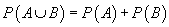If the events are not mutually exclusive (that is, they share some elements in common), then the probability of their union is sum of the individual probabilities minus the probability of all elements in common (this is just the intersection of A and B).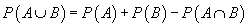This formula is actually a more general expression of the preceding formula. Fourth and finally, the probability of an event E is equal to unity minus the probability of the event's complement, EC. This statement simply combines the facts that E and EC are mutually exclusive but span the entire sample space S and that the probability of S is unity. Thus, using the rules above,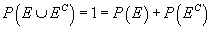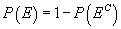Although these rules and concepts may seem somewhat esoteric, they are indeed helpful in discussing probability as it relates to statistics. The following practice problems will help you apply these ideas to practical problems and situations.

Practice Problem: For a random experiment involving the roll of a 10-sided die, what is the probability that the outcome will be between 1 and 10 inclusive?

Solution: The sample space for this experiment (assuming the die is labeled in the standard manner) is {1, 2, 3, 4, 5, 6, 7, 8, 9, 10}. Because the event for which the outcome of a roll is between 1 and 10 (inclusive) spans the sample space, the probability must simply be unity.

Practice Problem: Given a standard deck of 52 playing cards, what is the probability that a card pulled from the deck is either an ace or a spade?

Solution: This problem forces you to apply several different aspects of statistics. First, note that the sample space has 52 elements (one for each card), and the relative frequency (and therefore probability) for selecting any particular card is 1/52.

The problem defines two events, which we will call A and P. Event A is the selection of an ace, and event P is the selection of a spade. Although you may realize already that A and P are not mutually exclusive events, let's write out the two sets to illustrate. The notation used below is the value of the card (A for ace, for example) followed by the suit of the card (S for spades, for example).

A = {AD, AS, AC, AH}

P = {AS, 2S, 3S, 4S, 5S, 6S, 7S, 8S, 9S, 10S, JS, QS, KS}

Note that one element (outcome) is shared between the two sets. Let's now write the probability formula for the union of A and P, which is the probability that the card selected is either a spade or an ace.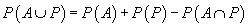We know by looking at the sets written out above that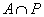is {AS}. Then,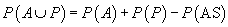Now, we must calculate these probabilities. First, we know that P(AS) is simply the probability that the selected card is the ace of spades--this is just 1/52. The relative frequency (and therefore probability) of selecting an ace is 4/52 = 1/13. The relative frequency (and therefore probability) of selecting a spade is 13/52 = 1/4. Let's use these numbers to calculate the probability that a random drawing of a card yields either an ace or a spade: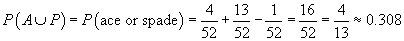Of course, a simpler approach would simply be to find the relative frequency of aces and spades (there are 16 such cards in a deck)--again, this is just 0.308. The solution above, however, illustrates the use of the concepts presented in this article. Other problems may not always be as easily solved.